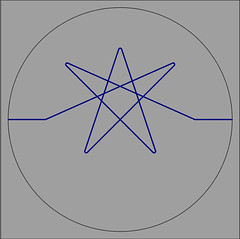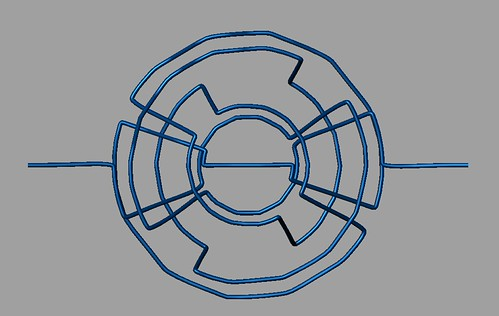## The Link of a Divide

You can view$S^3$ as the set of points${(x,v) | x in mathbb{R}^2, v in T_x mathbb{R}^2, ||x||^2 + ||v||^2 = 1}.$
So basically this would be the unit tangent bundle over the unit disk but you scale down the vectors as you get further from the origin. Over each circle about the origin of radius$r$ for$0 you get a torus separating$S^3$ into two solid tori as in an earlier post. At each$r=0$ and$r=1$ you get just a circle.

If you draw a properly immersed smooth 1-manifold in the unit disk (with only transveral self-intersections), then at each point away from the boundary and the origin its tangent line picks out two points in this model of$S^3$. At the boundary and the origin, it picks out just one point.Since these points vary smoothly you get a 1-manifold in$S^3$, a knot or a link. The immersed 1-manifold is called a divide and the links of a divide have many nice properties as A’Campo has shown.One can piece together what link is obtained from a divide, but Hirasawa has spelled it out quite nicely in “Visualization of A’Campo’s ﬁbered links and unknotting operation” (in Topology and its Applications).
Yamada got me interested in these things since he’s been showing that Berge’s knots (or their mirrors) can be obtained this way, and perhaps in a particular sort of way. He’s got a preprint that contains an overview of his work.

The new version of Mathematica has ramped up its interactivity and visualizations. I took it for a test spin with my sunday driver programming skillz at the wheel. Fortunately through the magic of comp.soft-sys.math.mathematica David Park helped me out. I’ve got a polygonal version of these divides done as a Mathematica Demonstration: A’Campo’s Link of a Divide

Here’s a pic of it, but Flickr requests it link back to them.You only need to download their free player to run this thing.
The Mathematica Demonstrations wouldn’t do exactly what I intended, hence the extra slider to change the viewpoint. If you have Mathematica 6, below is code that works a bit better.
I used this version to export a .dxf of the knot that I imported into Rhino3D and tweaked to produce the pictures at the beginning.

By all means, if you can make this code or the demonstration run faster, better, stronger, then I invite you to do so. Here it is:

Module[
(*The variables and initial input*)
{
(*Primary dynamic variables*)

mvpts = {{3.0, 0.68}, {1.34, -0.25}, {2.06,
0.97}, {2.64, -0.18}, {1.1, 0.81}},
(*(({2,0}+#)&/@Table[{i/3,0},{i,-2,
2}]),*)
(*Dependent dynamic variables*)
pts,
(*Other variables*)
calcAll,
yyy = {0, 1, 0},
ptangles,
ptsrotone,
ptsrottwo,
seg, arc, fxn
},
(*Computations*)

calcAll[movingpts_] := (pts =
Join[{{5, 0}, {3, 0}},
Reverse[movingpts], {{1, 0}, {0, 0}, {1, 0}},
movingpts, {{3, 0}, {5, 0}}];
ptangles =
Table[(If[pts[[i + 1]][] < pts[[i]][], -1, +1])
VectorAngle[{1, 0}, pts[[i + 1]] – pts[[i]]], {i, 1,
Length[pts] – 1}];
ptsrotone =
Table[RotationTransform[ptangles[[i]], yyy][
Append[pts[[i]], 0]], {i, 1, Length[ptangles]}];
ptsrottwo =
Table[RotationTransform[ptangles[[i]], yyy][
Append[pts[[i + 1]], 0]], {i, 1, Length[ptangles]}];
seg[k_, t_] :=
ptsrotone[[
k]] + (ptsrottwo[[k]] – ptsrotone[[k]]) (t –
2 k)(*this should run from t=2k to t=2k+1*);
arc[k_, t_] :=

RotationTransform[
Mod[ptangles[[k + 1]] – ptangles[[k]],
2 Pi, -Pi] (t – (2 k + 1)), yyy][
ptsrottwo[[k]]](*this should run from t=2k+1 to t=2k+2*);
fxn = Piecewise[
Table[If[
EvenQ[k] == True, {seg[k/2, t],
k <= t && t <= k + 1}, {arc[(k – 1)/2, t],
k <= t && t {{1, 3}, {-1, 1}}, ImageSize -> 200]],
Dynamic@
ParametricPlot3D[fxn, {t, 2, 2 Length[pts] – 1},
PlotStyle -> Thickness[.02],
ColorFunction ->
Function[{x, y, z, t}, ColorData[“RedBlueTones”][t]],
Axes -> False, SphericalRegion -> True, RotationAction -> Clip,
ImageSize -> 350, Boxed -> False, BoxRatios -> {1, 1, 1}]}]
]
]

~ by Ken Baker on October 26, 2008.